# Cross section (geometry)

For cross-sections in architecture and engineering, see Multiview orthographic projection § Cross-section.

In geometry and science, a cross section is the intersection of a body in three-dimensional space with a plane, or the analog in higher-dimensional space. Cutting an object into slices creates many parallel cross sections. A cross section of three-dimensional space that is parallel to two of the axes is a contour line; for example, if a plane cuts through mountains of a raised-relief map parallel to the ground, the result is a contour line in two-dimensional space showing points of equal elevation.

## Conic sections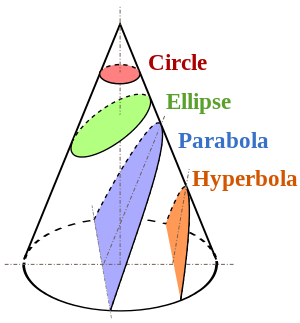Conic sectionscircles, ellipses, parabolas, and hyperbolas – are formed by cross-sections of a cone at various different angles, as seen in the diagram at left.

Any planar cross-section passing through the center of an ellipsoid forms an ellipse on its surface, which degenerates to a circle for sections perpendicular to a symmetry axis.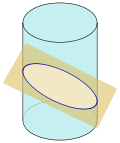Cylindric section.

A cross-section of a cylinder is a circle if the cross-section is parallel to the cylinder's base, or an ellipse with non-zero eccentricity (see diagram at right) if it is neither parallel nor perpendicular to the base. If the cross-section is perpendicular to the base it consists of two parallel line segments (not shown) unless it is just tangent to the cylinder, in which case it is a single line segment.

## Other mathematical examples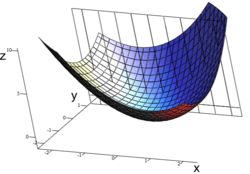A graph of z = x2 + xy + y2. For the partial derivative at (1, 1, 3) that leaves y constant, the corresponding tangent line is parallel to the xz-plane.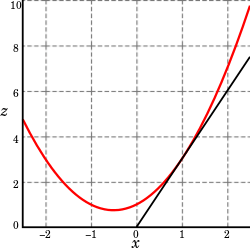A slice of the graph above showing the function in the xz-plane at y= 1

A cross section of a polyhedron is a polygon.

A cross section of a probability density function of two random variables in which the cross sectioning plane is at a fixed value of one of the variables is a conditional density function of the other variable (conditional on the fixed value defining the cross section). If instead the cross section is taken for a fixed value of the density, the result is an iso-density contour. For the normal distribution, these contours are ellipses.

A cross section can be used to visualize the partial derivative of a function with respect to one of its arguments, as shown at left. Suppose z = f(x, y). In taking the partial derivative of f(x, y) with respect to x, one can take a cross section of the function f at a fixed value of y to plot z solely against x; then the partial derivative with respect to x is the slope of the resulting two-dimensional graph.

In economics, a production function f(x, y) specifies the output that can be produced by various quantities x and y of inputs, typically labor and physical capital. The production function of a firm or a society can be plotted in three-dimensional space. If a cross section is taken parallel to the x, y plane, the result is an isoquant showing the various combinations of labor and capital usage that would result in the level of output given by the height of the cross section. Alternatively, if a cross section of the production function is taken at a fixed level of y—that is, parallel to the x, z plane—then the result is a two-dimensional graph showing how much output can be produced at each of various values of usage x of one input combined with the fixed value of the other input y.

Also in economics, a cardinal or ordinal utility function u(w, v) gives the degree of satisfaction of a consumer obtained by consuming quantities w and v of two goods. If a cross section of the utility function is taken at a given height (level of utility), the two-dimensional result is an indifference curve showing various alternative combinations of consumed amounts w and v of the two goods all of which give the specified level of utility.

## Examples in science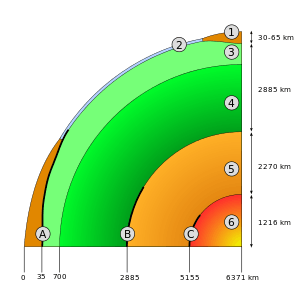Schematic view of the interior of Earth.
1. continental crust
2. oceanic crust
3. upper mantle
4. lower mantle
5. outer core
6. inner core
A: Mohorovičić discontinuity
B: core–mantle boundary (Gutenberg discontinuity)
C: Boundary of the inner core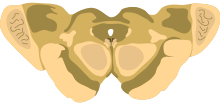Cross-section of the midbrain at the level of the superior colliculus.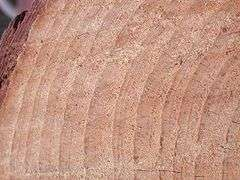Pinus taeda cross section showing annual rings, Cheraw, South Carolina.

In geology, the structure of the interior of a planet is often illustrated using a diagram of a cross section of the planet that passes through the planet's center, as in the cross section of Earth at right.

Cross sections are often used in anatomy to illustrate the inner structure of an organ, as shown at left.

A cross section of a tree trunk, as shown at left, reveals growth rings that can be used to find the age of the tree and the temporal properties of its environment.

## Area and volume

Cavalieri's principle states that solids with corresponding cross sections of equal areas have equal volumes.

The cross-sectional area () of an object when viewed from a particular angle is the total area of the orthographic projection of the object from that angle. For example, a cylinder of height h and radius r haswhen viewed along its central axis, andwhen viewed from an orthogonal direction. A sphere of radius r haswhen viewed from any angle. More generically,can be calculated by evaluating the following surface integral:whereis the unit vector pointing along the viewing direction toward the viewer,is a surface element with an outward-pointing normal, and the integral is taken only over the top-most surface, that part of the surface that is "visible" from the perspective of the viewer. For a convex body, each ray through the object from the viewer's perspective crosses just two surfaces. For such objects, the integral may be taken over the entire surface () by taking the absolute value of the integrand (so that the "top" and "bottom" of the object do not subtract away, as would be required by the Divergence Theorem applied to the constant vector field) and dividing by two:## Cross section of four-dimensional space

If a four-dimensional object passed through our three-dimensional space, we would see a three-dimensional cross-section of the four-dimensional object, such as a sphere that increased and then decreased in apparent size during the encounter.Wikimedia Commons has media related to Cross sections.